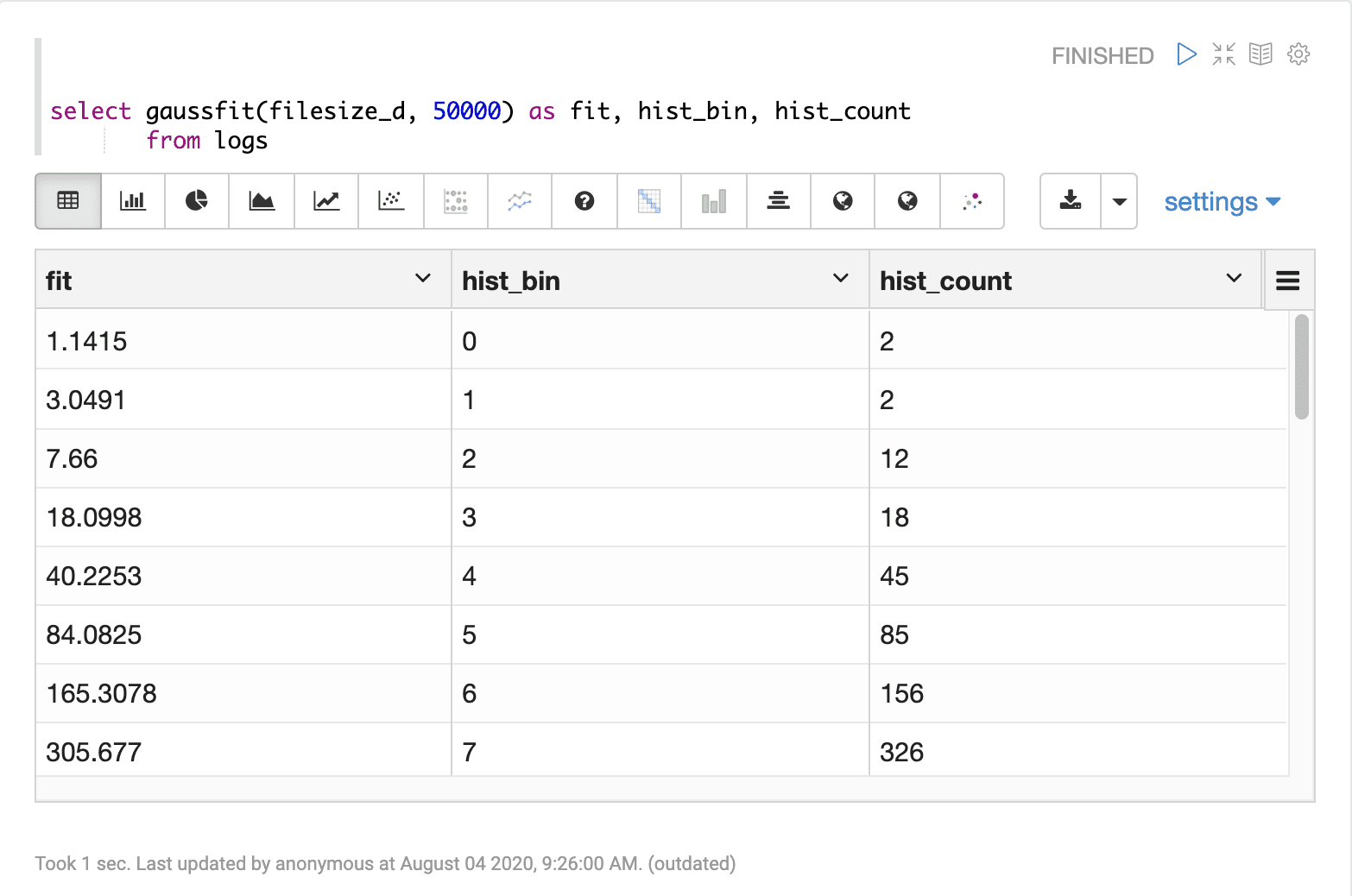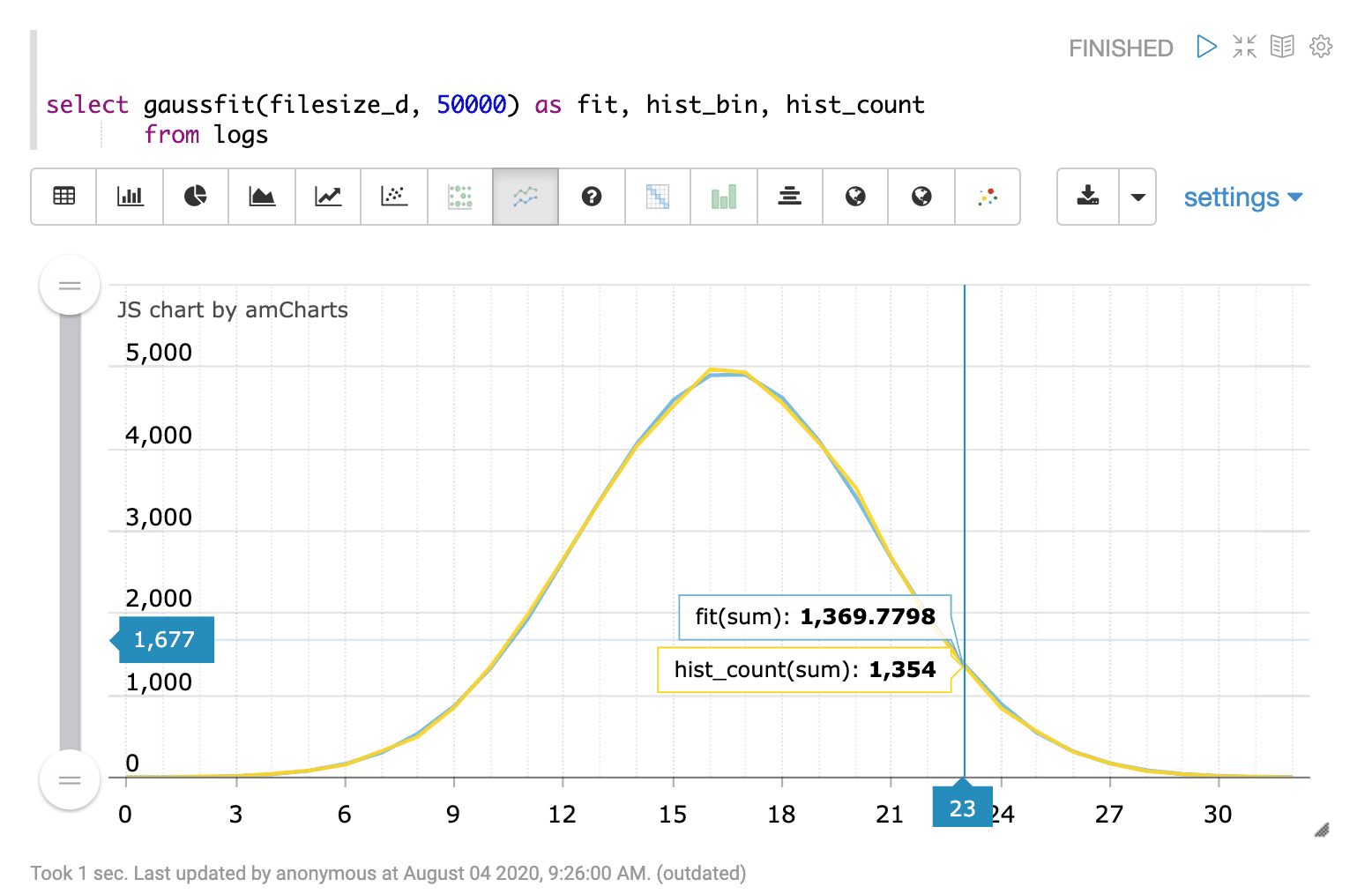# Gaussfit (gaussfit)

The `gaussfit` function fits a gaussian curve to a histogram constructed from a random sample drawn from a numeric field. The gaussfit function can be used to visualize how well the values in a numeric field fit a normal distribution. The `gaussfit` function takes two parameters:

1. the numeric field from which to draw the histogram

2. the sample size

## Sample syntax

``````select gaussfit(filesize_d, 50000) as fit,
hist_bin,
hist_count
from logs``````

## Result set

The gaussfit result set contains one record for each bin in the histogram drawn from the random sample. The `gaussfit` function returns the value of the fitted gaussian curve. The `hist_bin` and `hist_count` fields are also available in the gaussfit result set. The `hist_bin` field contains the histogram bin number and the `hist_count` field contains the count of samples in each bin.

Below is a sample result set in Apache Zeppelin:## Visualization

The gaussfit result set can be visualized by plotting the `hist_bin` column on the x-axis and the `fit` and the `hist_count` columns on the y-axis. The visualization belows shows the gaussfit result visualized in an Apache Zeppelin line chart: